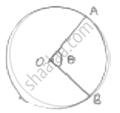Share

# The Perimeter of Certain Sector of Circle of Radius 5.6 M is 27.2 M. Find the Area of Sector. - CBSE Class 10 - Mathematics

ConceptAreas of Sector and Segment of a Circle

#### Question

The perimeter of certain sector of circle of radius 5.6 m is 27.2 m. Find the area of sector.

#### Solution𝜃 = angle subtended at centre

Radius (r) = 5.6m = OA ± OB

Perimeter of sector = 27.2 m

(AB arc length) + OA + OB = 27.2

⇒(theta/360^@× 2pir) + 5.6 + 5.6 ± 27.2

⇒(5.6 pitheta)/180^@+ 11.2 = 27.2

⇒ 5.6 ×22/7× 𝜃 = 16 × 180

⇒ 𝜃 =(16×180)/(0.8×22)= 163.64°

Area of sector =theta/360^@× pir^2 =163.64^@/360^@×22/7× 5.6 × 5.6

=163.64/180× 11 × 0.8 × 5.6

= 44.8 cm2

Is there an error in this question or solution?

#### APPEARS IN

Solution The Perimeter of Certain Sector of Circle of Radius 5.6 M is 27.2 M. Find the Area of Sector. Concept: Areas of Sector and Segment of a Circle.
S